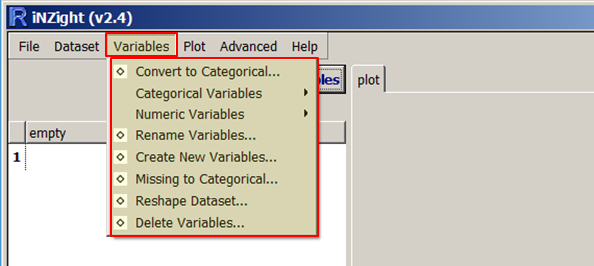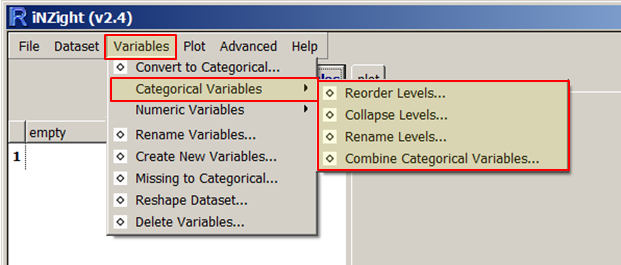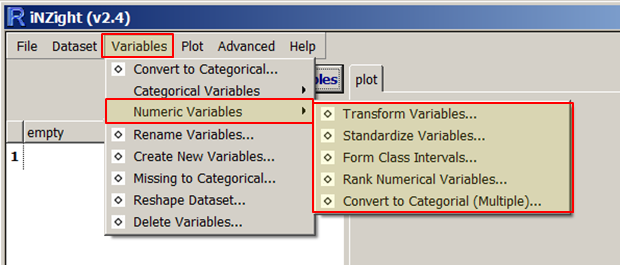This website is currently being developed. If you notice any problems (such as broken links, etc.), please report them.
< User Guides

# Variables

(Previously called 'Manipulate Variables')

iNZight assumes that data sets are in rows = cases by columns = variables format.

For example, the cases (also often called units) may be individual people and the columns = variables contain different types of "measures" on those people.

By default, if all the values of a variable are numbers, then that variable will be treated as as a numeric variable.

If any of the values contains even one alphabetic character, then the whole variable will be treated as a categorical variable (i.e., one that gives group membership). The one exception is the value `NA`, which is treated as a missing-value code in both numeric and categorical variables.Type-specific submenus: Categorical Variables, Numeric Variables

### Convert to Categorical

Creates a categorical version of a numeric variable.

### Rename Variables

Rename variables in the dataset. Especially useful for variables created by iNZight.

### Create New Variable

A very flexible facility for creating new variables from existing variables, essentially by doing arithmetic on them.

For example,

• `income = hours * payrate`
• `weight.diff = end.weight - begin.weight`
• `average.weight = ( begin.weight + endweight) / 2`

This new variable can take any valid R expression.

### Missing to Categorical

• Categorical variables: Any missing observations for the variable we be given a new level, `missing`. All others remain the same.

• Numeric variables: The variable will be converted into a categorical variable with two levels: `missing` and `observed`.

### Reshape Dataset

For use with data concerning a single variable in which different columns correspond to the measurements from different groups. Such data will be converted into the standard cases by variables format (example given in Reshape Dataset's dialog window).

### Delete Variables

Delete variables from the dataset.

## Categorical Variables### Reorder Levels

By default, the levels of a categorical variable are displayed in alpha-numeric order. This enables you to change from the default order of display to something more natural; e.g. from {"adolescent", "adult", "child", "elder"} to {"child", "adolescent", "adult", "elder"}.

### Collapse Levels

Combine levels within a categorical parent variable to make a new variable with a smaller number of levels. (The levels of a categorical variable are the set of unique, or distinct, values it takes).

### Rename Levels

Change the names of the levels of a categorical variable, e.g. from (ages) {"< 12", "12-17", "18-69", "> 69"} to {"child", "adolescent", "adult", "elder"}.

### Combine Categorical Variables

Take two categorical variables and create a new categorical variable whose levels are all combinations of those two (e.g., the combinations of ethnicity and gender).

## Numeric Variables### Transform Variables

Creates a new variable that is a transformed version of the parent variable. Transformations available are `log` (base `e` or base 10), exponential, square, square root and reciprocal.

### Standardise Variables

Create a standardised version of a variable (or z-score) by subtracting the mean of the variable from each value and dividing by its standard deviation.

### Form Class Intervals

Create a categorical variable whose levels are class intervals of a numeric variable.

For example, take `age` and create `age.f` with levels {"{0,20]", "(20,60]", "(60,110]"}.

### Rank Numerical Variables

Creates a new numeric variable containing the rank, or order of the selected variable. This is often used when the order of the values (but not the values themselves) is of interest.

For example, the variable `age = {15, 12, 19, 16}` would result in the new variable `age.rank = {2, 1, 4, 3}`.

### Convert to Categorical (Multiple)

This works the same as the previous "Convert to Categorical" tool, however it allows users to convert multiple variables at once.